# 13. Data Structures for Integers

In this chapter, we return to the problem of implementing an. The difference now is that we assume the elements stored in the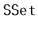are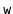-bit integers. That is, we want to implement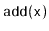,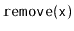, and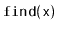where. It is not too hard to think of plenty of applications where the data--or at least the key that we use for sorting the data--is an integer.

We will discuss three data structures, each building on the ideas of the previous. The first structure, theperforms all threeoperations intime. This is not very impressive, since any subset of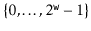has size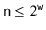, so that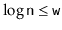. All the otherimplementations discussed in this book perform all operations intime so they are all at least as fast as a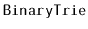.

The second structure, the, speeds up the search in aby using hashing. With this speedup, theoperation runs in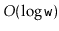time. However,andoperations in anstill take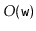time and the space used by anis.

The third data structure, the, uses an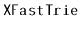to store only a sample of roughly one out of everyelements and stores the remaining elements in a standardstructure. This trick reduces the running time ofandtoand decreases the space to.

The implementations used as examples in this chapter can store any type of data, as long as an integer can be associated with it. In the code samples, the variableis always the integer value associated with, and the method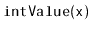convertsto its associated integer. In the text, however, we will simply treat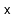as if it is an integer.

Subsections
opendatastructures.org